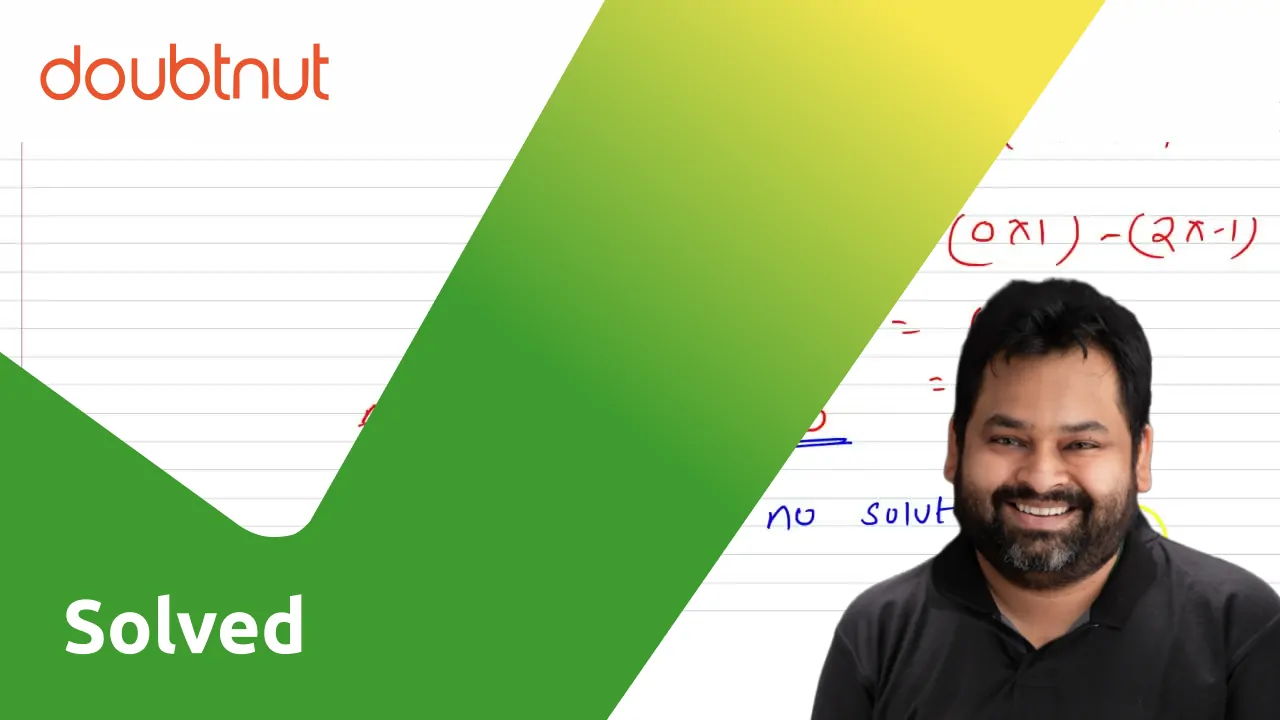Doubtnut App - Get 50 Lakh+ video solutions better than GoogleInstall Now

# Write the number of solution of the following system of equation. x+y+z=1 x+y+z=2 2x+3y+z=0Solution in Odia

Text Solution
Verified by Experts

## The correct Answer is:No solution

Video Solution